# SBI PO Prelims Reasoning Questions 2019 (Day-50)

Dear Aspirants, Our IBPS Guide team is providing new series of Reasoning Questions for SBI PO 2019 so the aspirants can practice it on a daily basis. These questions are framed by our skilled experts after understanding your needs thoroughly. Aspirants can practice these new series questions daily to familiarize with the exact exam pattern and make your preparation effective.

[WpProQuiz 6389]

### Click Here for SBI PO Pre 2019 High-Quality Mocks Exactly on SBI Standard

Direction (1-4): Study the following information carefully to answer the given questions:

Eight boxes numbered from 1-8 are arranged one above other but not necessarily in the same order. The positions of the boxes were numbered from 1-8 from bottom to top.

Box 7 is arranged immediately above Box 1. Two boxes are arranged between box 1 and box 4. One box is arranged between box 3 and box 8. Only the numbers of two boxes and their position are same. Box 7 is in the even numbered position. Box 3 is arranged above box 8. Even numbered boxes are arranged consecutively one above another but not in the same order. Box 1 is not placed at the bottommost position.

1) How many boxes are arranged between box 5 and box 2?

a) Two

b) One

c) None

d) Three

e) None of these

2) Four of the following five are alike in a certain way based on the arrangement and hence form a group. Which one of the following does not belong to that group?

a) Box 8

b) Box 3

c) Box 1

d) Box 6

e) Box 4

3) How many boxes are arranged above box 1?

a) One

b) Three

c) Five

d) Seven

e) None of these

4) If all the boxes are arranged in descending order from bottom to top then how many boxes are arranged in the same position?

a) None

b) Three

c) One

d) Two

e) None of these

Directions (5-6): Study the following information carefully and answer the below questions.

Ten persons are there in a family with three married couples. P is the father of C, who is the husband of J. A is the only daughter of C. Z is the mother of G and wife of P. G is married to I. H is the father of I. B is the only son of G. Q and A is child of J.

5) How is G related to P?

a) Son

b) Daughter

c) Son-in-law

d) Daughter-in-law

e) Cannot be determined

6) How is Q related to G?

a) Son

b) Nephew

c) Niece

d) Daughter

e) Cannot be determined

Directions (7-8): Study the following information carefully and answer the below questions.

Point A is 9m south of point B. Point G is 1m north of point D. Point E is 15m east of point D. Point F is 8m north of point E. The distance between point A and G is equal to point D and E. Point D is north of point B. Point C is 12m west of point B.

7) What is the shortest distance between point C and D?

a) √179m

b) 14m

c) 15m

d) 13m

e) Cannot be determined

8) If point X is 8m east of point A, then what is the sum of the shortest distance between point G and X and the shortest distance between point A and C?

a) √386m

b) 30m

c) Cannot be determined

d) 34m

e) 32m

9) How many such pairs of letters are there in the word ‘UNPREDICTABLE’ each of which has as many letters between them in the word as in the English alphabet?

a) Five

b) Two

c) Three

d) Seven

e) None of these

10) In a row of boys facing north direction, Kathir is 21st from the right end and Mani is 19th from the left end. Ragu is 8th to the left of Kathir and 5th to the right of Mani. How many boys are there in the row?

a) 53

b) 51

c) 52

d) 50

e) Cannot be determined

Directions (1-4):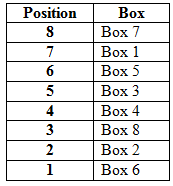Box 7 is arranged immediately above Box 1. Box 7 is in the even numbered position. Two boxes are arranged between box 1 and box 4. Box 1 is not placed at the bottommost position.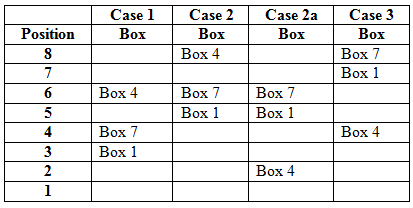Even numbered boxes are arranged consecutively one above another but not in the same order.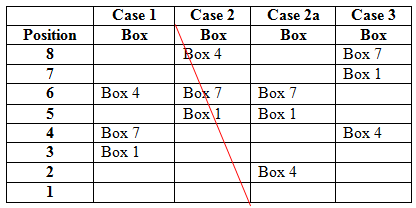Case 2 gets eliminated; since we can’t fix the positions of even numbered boxes consecutively.

One box is arranged between box 3 and box 8. Box 3 is arranged above box 8.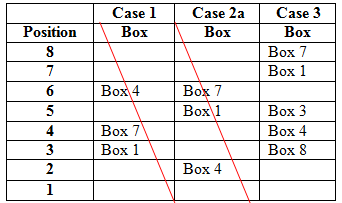Case 1 and Case 2a gets eliminated; since we can’t fix the positions of Box 8 and Box 3.

Only the numbers of two boxes and their position are same.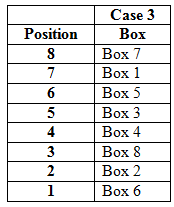Directions (5-6):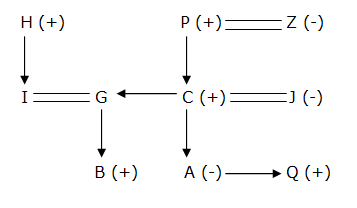The shortest distance between point C and D = √144 + √25 = √169 = 13m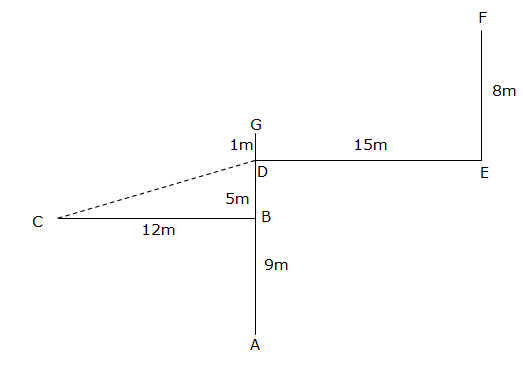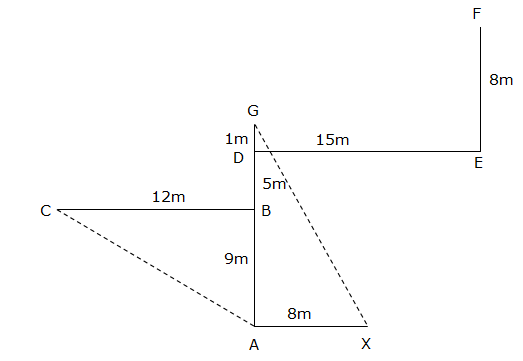Shortest distance between point G and X = √225 + √64 = √289 = 17m

Shortest distance between point A and C = √144 + √81 = √225 = 15m

So, 15 + 17 = 32m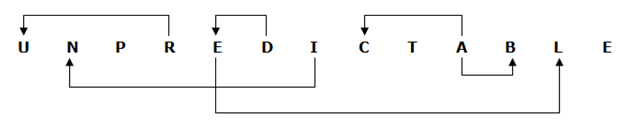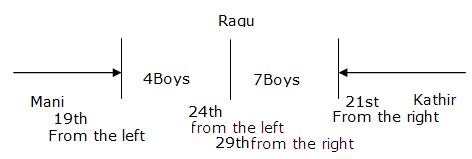Ragu rank from left 24th and from right 29th, here his/her rank is counted twice. So, from answer subtract one rank.

24+29=53

Ragu rank is twice so, 53-1=52.

SBI PO Prelims Reasoning Questions 2019 (Day-49)

SBI PO Prelims Reasoning Questions 2019 (Day-48)